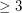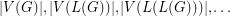# reconstruction

## Switching reconstruction conjecture ★★

Author(s): Stanley

Conjecture   Every simple graph on five or more vertices is switching-reconstructible.

Keywords: reconstruction

## Edge Reconstruction Conjecture ★★★

Author(s): Harary

Conjecture

Every simple graph with at least 4 edges is reconstructible from it's edge deleted subgraphs

Keywords: reconstruction

## Reconstruction conjecture ★★★★

Author(s): Kelly; Ulam

The deck of a graphis the multiset consisting of all unlabelled subgraphs obtained fromby deleting a vertex in all possible ways (counted according to multiplicity).

Conjecture   If two graphs onvertices have the same deck, then they are isomorphic.

Keywords: reconstruction

## Graham's conjecture on tree reconstruction ★★

Author(s): Graham

Problem   for every graph, we letdenote the line graph of. Given thatis a tree, can we determine it from the integer sequence?

Keywords: reconstruction; tree